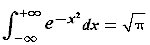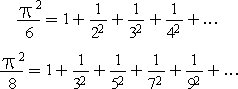# As Easy as Pi

The fascination with π is so universal that in 1998 film director Darren Aronofsky even created a psychological thriller with that title. Here I want to tell only a few more π stories that are perhaps less known outside the circle of true aficionados.
This post was published on the now-closed HuffPost Contributor platform. Contributors control their own work and posted freely to our site. If you need to flag this entry as abusive, send us an email.A door handle in the shape of pi is seen at the new National Museum of Mathematics in New York, Monday, Dec. 17, 2012. The museum is aimed at kids aged 8 to 13, and curators have given the place a playground feel. The 40 exhibits include a "wall of fire" made up of laser lights that teaches kids about geometry and a square-wheeled tricycle that still manages to produce a smooth ride thanks to a wavy track. (AP Photo/Seth Wenig)

There is probably no number in mathematics (with the possible exception of 0) that is more celebrated than the one equal to the ratio of a circle's circumference to its diameter. This number is denoted by the Greek letter π (pi). Pi is approximately equal to 3.14159, but its decimal representation neither ends nor settles into a repeating pattern. In fact, on Oct. 16, 2011, Alexander J. Yee and Shigeru Kondo completed the task of using a custom-built computer (shown in Fig. 1) for 371 days, to calculate π to 10 trillion digits! To appreciate this accuracy, let me note that if we wanted to express the radius of the observable universe in terms of the radius of the hydrogen atom, about 40 digits would have sufficed.Figure 1. The computer used by Alexander Yee and Shigeru Kondo to calculate π to 10 trillion digits (reproduced by permission from Alexander Yee)

The fascination with π is so universal that in 1998 film director Darren Aronofsky even created a psychological thriller with that title. Here I want to tell only a few more π stories that are perhaps less known outside the circle of true aficionados.

William Thomson (1824-1907), later known as Lord Kelvin, was the most famous physicist of his time and a superb mathematician. However, even excellent mathematicians have their own role models, and to Kelvin one such person was the French mathematician Joseph Liouville. Accordingly, in one of his lectures in Glasgow, after using the word "mathematician," Kelvin interrupted his own presentation and asked his students, "Do you know what a mathematician is?" And when the students didn't quite know what to answer, he wrote on the blackboard the following equation:This equation reflects the appearance of π in what is known as the "normal distribution," which is often used in probability theory. After writing it on the blackboard, Lord Kelvin declared, "A mathematician is one to whom that is as obvious as that twice two are four is to you. Liouville was a mathematician." Then he resumed his lecture.

A person to whom the value of π was apparently much less obvious was Edward Johnston Goodwin, a medical doctor of Solitude, Posey County, Ind. This physician-impersonating-a-mathematician authored a bill that was introduced in 1897 in the Indiana House of Representatives (by Rep. Taylor I. Record). In that bill Dr. Goodwin claimed that he had proved that "the ratio of the diameter and circumference is as five-fourths to four (in other words, that π = 3.2!); and because of these facts and the further fact that the rule in present use (that π = 3.1459...) fails to work both ways mathematically, it should be discarded as wholly wanting and misleading in practical applications." Believe it or not, this bill was approved in the House by a vote of 67 to nil. Fortunately, through the intervention of a professor of mathematics from Purdue University, the Indiana Senate decided to postpone any further action on the bill indefinitely.

Had that Indiana bill actually passed, it would have been quite interesting, given that π features in the equations that govern the behavior of the universe as a whole. In Einstein's theory of general relativity, the evolution of space-time is described by the following equation:

G = 8π G(T + ρ g)

The right-hand side represents the energy density associated with all forms of mass and energy in the universe (including the dark energy that propels the cosmic acceleration). The left-hand side of the equation represents the geometry of space-time. The equation as a whole reflects Einstein's revolutionary idea that the mass and energy contents of the universe determine the cosmic geometry. Consequently, the appearance of π in the equation is not surprising. Note that the adoption of Goodwin's value of π = 3.2 would have resulted in a different prediction for the evolution of the entire universe!

I have no doubt that π will continue to enthrall many generations to come. The fact is that mathematicians are still discovering new methods for calculating π. Let me end with two elegant and surprising such formulae:Close